# Riesz theorem(2)

(diff) ← Older revision | Latest revision (diff) | Newer revision → (diff)

Riesz's theorem on the representation of a subharmonic function: Ifis a subharmonic function in a domainof a Euclidean space,, then there exists a unique positive Borel measureonsuch that for any relatively compact setthe Riesz representation ofas the sum of a potential and a harmonic functionis valid:(1)

where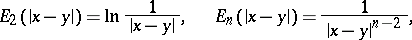and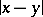is the distance between the points(see ). The measureis called the associated measure for the functionor the Riesz measure.

If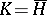is the closure of a domainand if, moreover, there exists a generalized Green function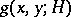, then formula (1) can be written in the form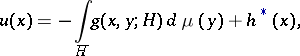(2)

whereis the least harmonic majorant ofin.

Formulas (1) and (2) can be extended under certain additional conditions to the entire domain(see Subharmonic function, and also , ).

Riesz's theorem on the mean value of a subharmonic function: Ifis a subharmonic function in a spherical shell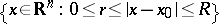, then its mean value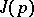over the area of the sphere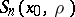with centre atand radius,, that is,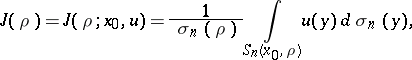whereis the area of, is a convex function with respect toforand with respect to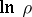for. Ifis a subharmonic function in the entire ball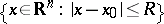, then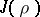is, furthermore, a non-decreasing continuous function with respect tounder the condition that(see ).

Riesz's theorem on analytic functions of Hardy classes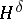,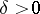: Ifis a regular analytic function in the unit disc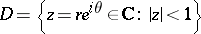of Hardy class,(see Boundary properties of analytic functions; Hardy classes), then the following relations hold: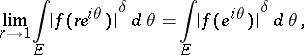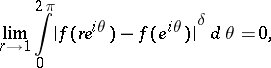whereis an arbitrary set of positive measure on the circle, and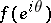are the boundary values ofon. Moreover,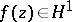if and only if its integral is continuous in the closed disc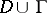and is absolutely continuous on(see ).

Theorems 1)–3) were proved by F. Riesz (see , ).

How to Cite This Entry:
Riesz theorem(2). Encyclopedia of Mathematics. URL: http://encyclopediaofmath.org/index.php?title=Riesz_theorem(2)&oldid=12058
This article was adapted from an original article by E.D. Solomentsev (originator), which appeared in Encyclopedia of Mathematics - ISBN 1402006098. See original article